# VAE classification

Hi!
Inspired by the SSVAE (The Semi-Supervised VAE — Pyro Tutorials 1.8.4 documentation) I am building a supervised VAE (I have also unsupervised and semisupervised versions). I am currently puzzled because my latent representations cluster according to the binary classification, however, the NN classifier (no matter what architecture) is incapable of generating the right classifications.

I would like some confirmation that the current architecture (here given over a dummy example) makes sense. I am worried about the correct use of the plates and the use of enumeration for discrete variables inference.
May you also please confirm that the masking over some positions across some positions in the sequence is valid? True means use this position to compute the likelihood, right? I am doubting everything at this point hehe

``````import torch
import torch.nn as nn
import pyro
import pyro.distributions as dist
from pyro.infer import config_enumerate,TraceEnum_ELBO,SVI
N = 50
L = 15
aa_types = 22
X = torch.randint(0,20,(N,L,aa_types)) #sequences
Y = torch.randint(0,1,(N,)) #labels
hidden_dim= 30
z_dim = 5
learning_type = "supervised"
class VAE_classifier(nn.Module):
def __init__(self):
super(VAE_classifier, self).__init__()
self.beta = 1 #no scaling right now
self.gru_hidden_dim = self.hidden_dim*2
self.aa_types = aa_types
self.max_len = L
self.z_dim = z_dim
self.num_classes = 2
self.learning_type = learning_type
self.encoder = RNN_encoder(self.aa_types,self.max_len,self.gru_hidden_dim,self.z_dim,self.device)
self.encoder_guide = RNN_encoder(self.aa_types,self.max_len,self.gru_hidden_dim,self.z_dim,self.device)
self.decoder = RNN_decoder(self.aa_types,self.seq_max_len,self.gru_hidden_dim,self.aa_types,self.z_dim ,self.device)
self.classifier_model = MLP(self.z_dim,self.max_len,self.hidden_dim,self.num_classes,self.device)
self.logsoftmax = nn.LogSoftmax(dim=-1)
pyro.module("vae_model", self)
batch_size = batch_sequences.shape
confidence_mask = (batch_confidence_scores[..., None] > 0.7).any(-1)
init_h_0_encoder = self.h_0_MODEL_encoder.expand(self.encoder.num_layers * 2, batch_sequences.shape,self.gru_hidden_dim).contiguous()

z_mean,z_scale = self.encoder(batch_sequences,init_h_0_encoder)
with pyro.poutine.scale(scale=self.beta):
with pyro.plate("plate_latent", batch_size,device=self.device):
latent_space = pyro.sample("latent_z", dist.Normal(z_mean, z_scale).to_event(1))  # [n,z_dim]

latent_z_seq = latent_space.repeat(1, self.max_len).reshape(batch_size, self.max_len, self.z_dim)

with pyro.plate("plate_class_seq",batch_size,dim=-1,device=self.device):
class_logits = self.classifier_model(latent_space)
class_logits = self.logsoftmax(class_logits)
assert class_logits.shape == (batch_size, self.num_classes)
if self.learning_type == "semisupervised":
elif self.learning_type == "supervised":
pyro.sample("predictions", dist.Categorical(logits=class_logits).to_event(1),obs=batch_true_labels,infer={'enumerate': 'parallel'})
else:
pyro.sample("predictions", dist.Categorical(logits=class_logits).to_event(1))

init_h_0_decoder = self.h_0_MODEL_decoder.expand(self.decoder.num_layers * 2, batch_size,self.gru_hidden_dim).contiguous()
with pyro.poutine.mask(mask=batch_mask): ## DOUBT: I want to mask some positions in the sequence, is this enough? I checked the trace and seemed correct
with pyro.plate("data_len",self.max_len,device=self.device):
with pyro.plate("data", batch_size,device=self.device):
sequences_logits = self.decoder(latent_z_seq,init_h_0_decoder)
sequences_logits = self.logsoftmax(sequences_logits)

pyro.module("vae_guide", self)
batch_size = batch_sequences.shape
confidence_mask = (batch_confidence_scores[..., None] < 0.7).any(-1) #now we try to predict those with a low confidence score

init_h_0 = self.h_0_GUIDE.expand(self.encoder_guide.num_layers * 2, batch_size,self.gru_hidden_dim).contiguous()  # bidirectional
with pyro.poutine.scale(scale=self.beta):
with pyro.plate("plate_latent", batch_size,device=self.device): #dim = -2
z_mean, z_scale = self.encoder_guide(batch_sequences, init_h_0)
assert z_mean.shape == (batch_size, self.z_dim), "Wrong shape got {}".format(z_mean.shape)
assert z_scale.shape == (batch_size, self.z_dim), "Wrong shape got {}".format(z_scale.shape)
latent_space = pyro.sample("latent_z", dist.Normal(z_mean,z_scale).to_event(1))  # [z_dim,n]

if self.learning_type in ["semisupervised","unsupervised"]:
with pyro.plate("plate_class_seq", batch_size, dim=-1, device=self.device):
class_logits = self.classifier_guide(latent_space)
class_logits = self.logsoftmax(class_logits)
assert class_logits.shape == (batch_size,self.num_classes)
if self.learning_type == "semisupervised":
else: #unsupervised
pyro.sample("predictions", dist.Categorical(logits=class_logits).to_event(1))

guide = config_enumerate(VAE_classifier.guide)
svi = SVI(VAE_classifier.model, guide, optimizer, TraceEnum_ELBO(max_plate_nesting=1))
``````

The encoder and decoders are just simple RNN + FCL . The NN architecture of the classifier is a simple MLP, since I tried more complex architectures and it would only predict one class (I also think it does not need it, given the nice clustering). I do not think is a hyperparameter issue either.

I want to confirm that the usage of config_enumerate, TraceEnum_ELBO, the plates and the masks makes sense, or what should I look into? Or should I use Trace_ELBO()?

Are the unsupervised and semisupervised modes making sense?

It is strange to me that the latent representations cluster perfectly and the NN classifier cannot figure out the right prediction ( I have checked my code for errors unrelated to the modelling and could not find bugs (for now)).

Note that the supervised model outperforms the unsupervised, therefore the values of the observed labels are being used to infer the latent random variables.

That is why I think there is something off with my approach to binary classification of the latent space,

Hi @artistworking . Not sure what you are trying to achieve but there are several things that jump out to me in your code:

1. I don’t think you need an encoder for `z` in the model. Typically you can use a standard Normal distribution `Normal(0, 1)`.
2. Enumeration is only used for latent variables. Since `predictions` is observed in your model it cannot be enumerated.
3. You only need one plate for both `z` and observed `predictions`. In general you should think through what plates you need in your model, looks like there are too many of them.
4. You cannot have observed sites in the guide.

I would recommend writing the code only for the supervised model to make the logic in your code easier and also make sure that your code matches the probabilistic model you are trying to build.

Yes, I am mostly working with the supervised approach but I would also like to know the correct approach for a semi-supervised and unsupervised approach.

1. I tried without the encoder and a simple N(0,1) and that just lead into nan values. Thereafter, I tried a “smart” prior for z_mean and z_std in the model but it was not good either (no nan values but non sensical results). The NN encoder in the model has worked pretty well.

2. Ok, yeah, I still have a mess in my head with the enumeration thing. Understood, no enumeration for observations.

3. Ok, yes, I guess I am using quite some plates … That could be the root cause, somehow I am making things independent of each other. I will have a look at it carefully.

4. I am aware you cannot have observations in the guide, but for the semi-supervised approach … should I just remove the observations and let the solely the mask point out which values are the latent random variables? I do not know why I thought by adding the observations argument I would inform the guide which ones are observed and which ones not…ops.

5. Once again, to sum up, for latent variables (unobserved) that are discrete, the usage of config_enum for the guide and parallel enumeration is correct, or? (so basically just for the unsupervised and semisupervised approaches)

6. What about TraceEnum_ELBO(), is it just recommended when we need to enumerate sites? It was not clear to me from the doc string of the class

Finally, in your opinion, designing a model where, I infer the latent representation of the sequences and from that latent representation I make a binary classification should work if done correctly? (specially since I see signal from the latent space representation?). I mean, I am aware VAE are mostly used as generative models, but why not as classifiers? There is not much literature on VAE as classifiers, that I have seen, that is why I am asking.

Thanks again!

I still think you should use `Normal(0, 1)` prior because the prior should be uninformed of your data.

My understanding is you are trying to build something like this:

• Prior `p(z)` - `Normal(0, 1)` (this is in the model)
• Likelihood for observed classes `p(y | z)` - `Bernoulli(neural_net(z))` (this is in the model)
• Guide (approx. posterior) `q(z | x)` - `Normal(encoder_loc(x), encoder_scale(x))` (this is in the guide)

I don’t see a reason why it shouldn’t work.

I don’t think you need any of enumeration and masking in your code. And you need just one `data` plate for both `z` and `y`.

1. Yes.
2. Yes, use `TraceEnum_ELBO` when you need to enumerate sites.

@ordabayev Yes, most likely you are right and my plate organization mess might have impeded the usage of the uninformative prior. I have reorganized the plates (see below) and that allows me to use a prior` p(z) - N(0,1)` without generating nan values, although the signal (latent space clustering) is not there at all. Is it bad to use a prior over z?

I am half way reorganizing my plates, therefore it looks something like this (I have not completely decided if I want to declare conditional independence over the sequence elements though, but let’s assume yes for now, therefore the “plate_len”)):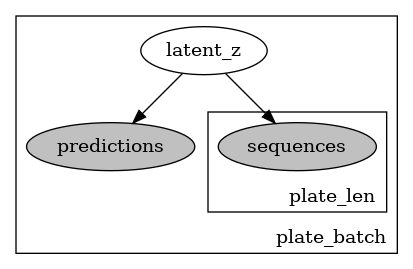a) However, I cannot a mask anymore over the sequence observations. I am reading across the forum and it seems like the issue has to do with masks not being broadcastable with event_dim?

I mean if my sequence and my mask are something like:

``````X = torch.randint(0,20,(N,L,aa_types)) #sequences
``````

b) If I mask them over the length dimension (dim = 1) , do they have to be inferred by the guide then? (I mean that this is not done automatically and I need to explicitely state it)

c) I also please would like to know if there is any differences among `pyro.poutine.mask()`, using the flag `obs_mask` in a distribution or using `.mask()` over a distribution. These are the documentations

Convenient wrapper of `MaskMessenger`

Given a stochastic function with some batched sample statements and masking tensor, mask out some of the sample statements elementwise.

Parameters

• fn – a stochastic function (callable containing Pyro primitive calls)
• mask (torch.BoolTensor) – a `{0,1}`-valued masking tensor (1 includes a site, 0 excludes a site)

Returns

stochastic function decorated with a `MaskMessenger`

obs_mask (bool or Tensor) – Optional boolean tensor mask of shape broadcastable with `fn.batch_shape`. If provided, events with mask=True will be conditioned on `obs` and remaining events will be imputed by sampling. This introduces a latent sample site named `name + "_unobserved"` which should be used by guides.

Parameters

mask (bool or torch.Tensor) – A boolean or boolean valued tensor.

Returns: A masked copy of this distribution.

Return type: `MaskedDistribution`

The code looks something like:

``````import torch
import torch.nn as nn
import pyro
import pyro.distributions as dist
from pyro.infer import config_enumerate,TraceEnum_ELBO,SVI,Trace_ELBO
N = 50
L = 15
aa_types = 22
X = torch.randint(0,20,(N,L,aa_types)) #sequences
Y = torch.randint(0,1,(N,)) #labels
hidden_dim= 30
gru_hidden_dim = 50
z_dim = 5
learning_type = "supervised"
class VAE_classifier(nn.Module):
def __init__(self):
super(VAE_classifier, self).__init__()
self.beta = 1 #no scaling right now
self.gru_hidden_dim = self.hidden_dim*2
self.aa_types = aa_types
self.max_len = L
self.z_dim = z_dim
self.num_classes = 2
self.encoder = RNN_encoder(self.aa_types,self.max_len,self.gru_hidden_dim,self.z_dim,self.device)
self.encoder_guide = RNN_encoder(self.aa_types,self.max_len,self.gru_hidden_dim,self.z_dim,self.device)
self.decoder = RNN_decoder(self.aa_types,self.seq_max_len,self.gru_hidden_dim,self.aa_types,self.z_dim ,self.device)
self.classifier_model = MLP(self.z_dim,self.max_len,self.hidden_dim,self.num_classes,self.device)
self.logsoftmax = nn.LogSoftmax(dim=-1)
pyro.module("vae_model", self)
batch_size = batch_sequences.shape
confidence_mask = (batch_confidence_scores[..., None] > 0.7).any(-1)
init_h_0_encoder = self.h_0_MODEL_encoder.expand(self.encoder.num_layers * 2, batch_sequences.shape,self.gru_hidden_dim).contiguous()

z_mean,z_scale = self.encoder(batch_sequences,init_h_0_encoder)
with pyro.poutine.scale(scale=self.beta):
with pyro.plate("plate_batch", batch_size,device=self.device):
latent_space = pyro.sample("latent_z", dist.Normal(z_mean, z_scale).to_event(1))  # [n,z_dim]
latent_z_seq = latent_space.repeat(1, self.max_len).reshape(batch_size, self.max_len, self.z_dim)
class_logits = self.classifier_model(latent_space)
class_logits = self.logsoftmax(class_logits)
assert class_logits.shape == (batch_size, self.num_classes)
if self.learning_type == "semisupervised":
elif self.learning_type == "supervised":
pyro.sample("predictions", dist.Categorical(logits=class_logits).to_event(1),obs=batch_true_labels)
else:
pyro.sample("predictions", dist.Categorical(logits=class_logits).to_event(1))

init_h_0_decoder = self.h_0_MODEL_decoder.expand(self.decoder.num_layers * 2, batch_size,self.gru_hidden_dim).contiguous()
with pyro.plate("plate_len",self.max_len,device=self.device):
sequences_logits = self.decoder(latent_z_seq,init_h_0_decoder)
sequences_logits = self.logsoftmax(sequences_logits)
pyro.sample("sequences",dist.Categorical(logits=sequences_logits).to_event(1),obs=batch_sequences)

pyro.module("vae_guide", self)
batch_size = batch_sequences.shape
confidence_mask = (batch_confidence_scores[..., None] > 0.7).any(-1) #now we try to predict those with a low confidence score

init_h_0 = self.h_0_GUIDE.expand(self.encoder_guide.num_layers * 2, batch_size,self.gru_hidden_dim).contiguous()  # bidirectional
with pyro.poutine.scale(scale=self.beta):
with pyro.plate("plate_batch", batch_size,device=self.device): #dim = -2
z_mean, z_scale = self.encoder_guide(batch_sequences, init_h_0)
assert z_mean.shape == (batch_size, self.z_dim), "Wrong shape got {}".format(z_mean.shape)
assert z_scale.shape == (batch_size, self.z_dim), "Wrong shape got {}".format(z_scale.shape)
latent_space = pyro.sample("latent_z", dist.Normal(z_mean,z_scale).to_event(1))  # [z_dim,n]

if self.learning_type in ["semisupervised","unsupervised"]:
class_logits = self.classifier_guide(latent_space)
class_logits = self.logsoftmax(class_logits)
assert class_logits.shape == (batch_size,self.num_classes)
if self.learning_type == "semisupervised":
else: #unsupervised
pyro.sample("predictions", dist.Categorical(logits=class_logits).to_event(1),infer={'enumerate': 'parallel'})

if learning_type in ["unsupervised","semisupervised"]:
guide = config_enumerate(VAE_classifier.guide)
loss = TraceEnum_ELBO()
else:
guide = VAE_classifier.guide
loss = Trace_ELBO()
svi = SVI(VAE_classifier.model, guide, optimizer, loss)
``````

Thanks again!!!@ordabayev I am asking about the mask because now that I have placed everything under the same plate I cannot get over this error while computing the model trace (Note that N = 100 and L =10 in this error)

File “/home/…/miniconda3/lib/python3.8/site-packages/pyro/poutine/trace_struct.py”, line 242, in compute_log_prob
File “/home/…/miniconda3/lib/python3.8/site-packages/pyro/distributions/util.py”, line 319, in scale_and_mask
RuntimeError: The size of tensor a (10) must match the size of tensor b (100) at non-singleton dimension 1

which refers to the log probability computation of the sequences, since I declare the length dimension as dependent and the mask somehow does not capture that

` pyro.sample("sequences",dist.Categorical(logits=sequences_logits).to_event(1),obs=batch_sequences)`

Note: I have tried to use

` pyro.deterministic ("mask",batch_mask, event_dim=1)`

to overcome the issue with no luck

Thanks!

Ok, I fixed the model like this:

``````   def model(self,batch_sequences,batch_mask,batch_true_labels,batch_confidence_scores):
pyro.module("vae_model", self)
batch_size = batch_sequences.shape
confidence_mask = (batch_confidence_scores[..., None] > 0.7).any(-1)
init_h_0_encoder = self.h_0_MODEL_encoder.expand(self.encoder.num_layers * 2, batch_sequences.shape,self.gru_hidden_dim).contiguous()

z_mean,z_scale = self.encoder(batch_sequences,init_h_0_encoder)
with pyro.poutine.scale(scale=self.beta):
with pyro.plate("plate_batch", dim=-1,device=self.device):
latent_space = pyro.sample("latent_z", dist.Normal(z_mean, z_scale).to_event(1))  # [n,z_dim]
latent_z_seq = latent_space.repeat(1, self.max_len).reshape(batch_size, self.max_len, self.z_dim)
class_logits = self.classifier_model(latent_space)
class_logits = self.logsoftmax(class_logits)
assert class_logits.shape == (batch_size, self.num_classes)
if self.learning_type == "semisupervised":
elif self.learning_type == "supervised":
pyro.sample("predictions", dist.Categorical(logits=class_logits).to_event(1),obs=batch_true_labels)
else:
pyro.sample("predictions", dist.Categorical(logits=class_logits).to_event(1))

init_h_0_decoder = self.h_0_MODEL_decoder.expand(self.decoder.num_layers * 2, batch_size,self.gru_hidden_dim).contiguous()
with pyro.plate("plate_len",dim=-2,device=self.device):
sequences_logits = self.decoder(latent_z_seq,init_h_0_decoder)
sequences_logits = self.logsoftmax(sequences_logits)
pyro.sample("sequences",dist.Categorical(logits=sequences_logits),obs=batch_sequences)
``````

Still no good classification, hmmm… I have tried both to declare the positions in the sequence as dependant and independent

You can mask over event_dim using `.mask()` like this:

``````>>> d = dist.Normal(torch.zeros(3), torch.ones(3))
tensor(-1.8379)
``````

Not sure I understand what you mean by inferred here. But yeah, you have to explicitly mask in the guide as well.

The difference between `poutine.mask()` and `.mask()` is subtle. Below they have a similar effect:

``````# preferred way to mask over batch shape
pyro.sample("x", dist_x)
pyro.sample("y", dist_y)

# vs

``````

Two differences that I know of are 1) you can mask event dims with `.mask()` as I showed above and 2) you can use `poutine.mask()` in cases when you have model enumerated variables:

``````# x is enumerated (marginalized) in the model
# this will first marginalize `x` p(y) = sum_x [ p(x)p(y|x) ]
# and then mask contracted factor p(y)
x= pyro.sample("x", Categorical(...), infer={"enumerate": "parallel"})
pyro.sample("y", Normal(x, 1)). # y depends on x

# this will mask `y` distribution and then marginalize `x`
x= pyro.sample("x", Categorical(...), infer={"enumerate": "parallel"})
``````

`obs_mask` works as explained in the documentation in conjunction with the `obs` keyword argument.

@ordabayev Thanks a lot for the patience. Apparently, I made some more mistakes with my plates (see above ) that is why I could not use the mask as intended. I have made more changes to the code and therefore I have a few more questions:

a) I need to reassure the correct usage of the mask. If I have a a batch of size 3 sequences with lenght 6 such that: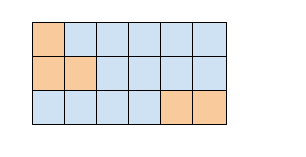where the orange positions are those to be ignored (padding) and we are only interested in reconstructing the blue ones. Then, in the model the orange sites are masked but they still have to be sampled in the guide? Even though we do not “care about them”? Such that:

``````def model():
....
sequences_logits = self.decoder(latent_z,init_h_0_decoder)
sequences_logits = self.logsoftmax(sequences_logits)
``````
``````def guide():
....
sequences_logits = self.decoder_guide(latent_z,init_h_0_decoder_guide)
sequences_logits = self.logsoftmax(sequences_logits)
``````

b) I though my “custom” sampling method was not correct so I switched to the Predictive class. The results are similar so far… My “custom” implementation of the sampling is something like (I keep the plates for structure):

``````def sample(self,batch_sequences,batch_mask,batch_true_labels,batch_confidence_scores,argmax=False):
""""""
batch_size = batch_sequences.shape
confidence_mask = (batch_confidence_scores[..., None] > 0.7).any(-1)  # now we try to predict those with a low confidence score

init_h_0_encoder = self.h_0_MODEL_encoder.expand(self.encoder.num_layers * 2, batch_size,self.gru_hidden_dim).contiguous()
z_mean,z_scale = self.encoder(batch_sequences,init_h_0_encoder)

with pyro.poutine.scale(scale=self.beta):
with pyro.plate("plate_batch",dim=-1):
latent_space = dist.Normal(z_mean, z_scale).sample()  # [n,z_dim]
latent_z_seq = latent_space.repeat(1, self.max_len).reshape(latent_space.shape, self.max_len, self.z_dim)
class_logits = self.classifier_model(latent_space, None)
class_logits = self.logsoftmax(class_logits)
if argmax:
predicted_labels = torch.argmax(class_logits, dim=1)
else:
predicted_labels = dist.Categorical(logits=class_logits).sample()
init_h_0_decoder = self.h_0_MODEL_decoder.expand(self.decoder.num_layers * 2, batch_size ,self.gru_hidden_dim).contiguous()  # bidirectional
#with pyro.plate("plate_len",dim=-2, device=self.device):  #Highlight: not to_event(1) and with our without plate over the len dimension
# Highlight: Forward network
sequences_logits = self.decoder(latent_z_seq, init_h_0_decoder)
sequences_logits = self.logsoftmax(sequences_logits)
reconstructed_sequences = dist.Categorical(logits= sequences_logits).sample()

return {"latent_space": latent_space,
"predicted_labels" : predicted_labels,
"reconstructed_sequences" : reconstructed_sequences}
``````

Is it wrong? Or missing something?

Some RESULTS

• It is important to note that I have a somewhat inbalanced dataset (~68% class 0), where the class 0 assignation is rather uncertain for a lot of cases (that is why I might move on to unsupervised or semi supervised approaches).
• Unfortunately, with the above mentioned fixes (except for sampling the masked sequences as in question a) which I have not implemented), the sequence reconstruction accuracies and the class prediction AUC are really bad. Note that I use the mode of the number of samples that I sample when plotting the accuracy or calculating AUC.
• The reconstruction accuracy stays steady throughout training, therefore it is not even trying.
• The class prediction accuracy increases for the training but reaches the 68% ceiling from class 0. It stays around 50% for the validation dataset.
• My gradients do not seem to vanish
• The error loss descends for the training dataset, but at some point it gives up for the validation.
• The only positive thing holding on right now is a possible grouping of the latent space that only occurs when the model is supervised (I have to make sure the unsupervised and semisupervised versions are correct in order to assume this).

See the results below for 250 epochs (the signal of the latent space structure appears quite soon but I train it longer):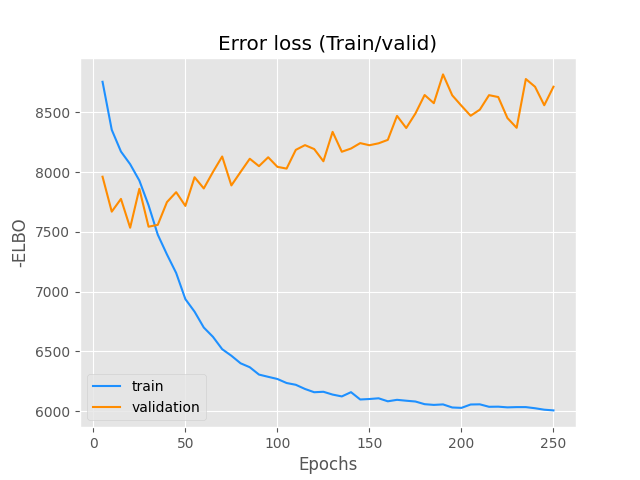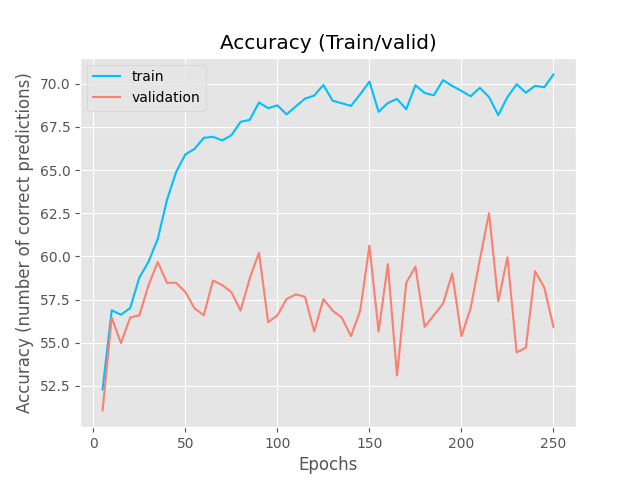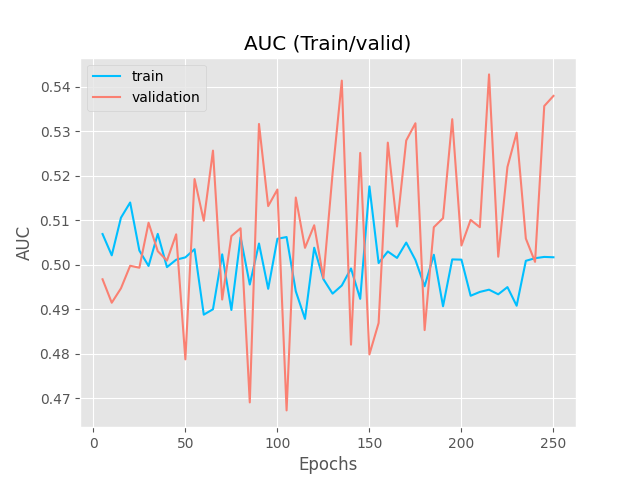It is obviously overfitting to the observed labels, but then , how is it possible that the classifier NN is so lost? Shouldn’t it also at least overfit?

In a):

• since you are already using `poutine.mask` you don’t need to mask the `Categorical` distribution with `.mask`
• you don’t need `pyro.sample` in the guide for sample sites that are observed in the model, i.e. `sequences` site.

Before dealing with masking (it can be tricky) I would recommend going through pyro tutorials to get a good grasp of how `pyro.sample` works, how `obs=` keyword works, how model/guide pair should be structured, how `pyro.sample` is different from `SomeDistribution.sample`, etc.

@ordabayev Thanks for the reply. I will review once again the documentation, but for now, are these statements correct? In very simple words:

`pyro.sample`, uses a stochastic function (such as a pyro distribution) to initiate the parameter store and the trace (graph structure that denotes the relationships of the pyro primitives, like pyro.sample) during inference. It operates according to the arguments (such as obs) given to pyro.sample and other details related to the inference algorithm.

`dist.sample()` performs sampling. Sampling returns a random variable by using high probable random numbers under the distribution’s PDF with the given parameter values (it works somehow along the lines of generating uniformly distributed pseudo random numbers and the using some “smart” and “efficient” method to reject those not falling within the desired PDF (i.e PCG Family of Random Number Generators, Ziggurat algorithm )) . Overall sampling and calculating the marginal log probability (PDF) of an observation under the model parameter(s) are the most common usages of pyro/torch distributions during optimization process.

Said that, it is the first time I am using the `masks` and I have started questioning everything.

P.S: I am reading this: Introduction to Pyro — Pyro Tutorials 1.8.4 documentation (which did not exist when I started with pyro, makes things easier. Amazing, wish I started something like that hehe). I will implement/plot more stuff!

@ordabayev I think I have improved the NN classifier with the above “fixes” and more sampling from the Predictive distribution (the AUC is not good though).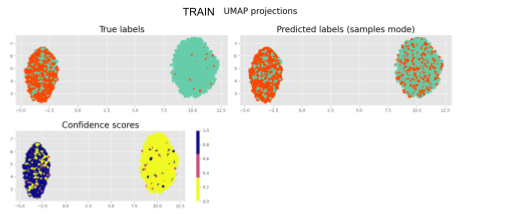However, my model mostly “shaping” the latent space by solely looking at the labels. Do you have any advice to improve the reconstruction of the sequences?

• I am looking into `poutine.scale` to boost up the influence of the reconstruction loss and KL combo. So, I guess I can just scale the down the classification loss with an “annealing factor” (similar to KL annealing) to decrease the ELBO (increase the -ELBO) and hopefully escape some local minima? Something like this?
``````def model():
.....
with pyro.poutine.scale(scale=annealing_factor):
pyro.sample("predictions",dist.Categorical(logits=class_logits).to_event(1),obs=batch_true_labels)

``````
• Or should I scale up the KL divergence? (lower KL higher -ELBO)

• Or scale up the reconstruction loss?

Would you recommend bothering with IAFS (Autoregressive NN) to improve the latent distribution of z in the guide? I have seen some articles ([2105.02027] Non-Autoregressive vs Autoregressive Neural Networks for System Identification) saying that they are just slower and do not bring anything to the table …

Any other advice is appreciated, thanks!